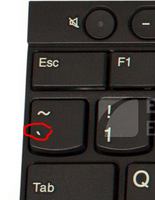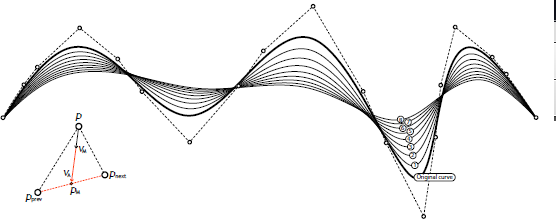# Could not convert Rhino.Geometry.Point3d object to a Point3d

Hello
I am a beginner of RhinoPython and learning through the book RhinoPythonPrimerRev3, Unfortunately. I encountered problems in the example exercises. the following is my code and return, please give me a hint, and where can I understand Different similar issues…
I have been troubled for a long time, because the information searched in the forum is incomplete.

thank you very much~

``````> import rhinoscriptsyntax as rs
>
> def smoothingvector(pt, prevpt, nextpt, s):
>     pm = (prevpt+nextpt)/2.0
>     va = rs.VectorCreate(pm, pt)
>     vm = rs.VectorScale(va, s)
>     return vm
>
>
> def smoothcurve(crv_id, s):
>     crv_pts = rs.CurvePoints(crv_id)
>     newcrv_pts = [  ]
>
>     for i in range(len(crv_pts)-1):
>         vm = smoothingvector(crv_pts[i],crv_pts[i-1],crv_pts[i+1],s)
>
>     knots = rs.CurveKnots(crv_id)
>     degree = rs.CurveDegree(crv_id)
>     weights = rs.CurveWeights(crv_id,0)
>     newcrv_id = rs.AddNurbsCurve(newcrv_pts, knots, degree, weights)
>     if newcrv_id: rs.DeleteObject(crv_id)
>     return newcrv_id
>
>
> def iterativeshortencurve():
>     crv_id = rs.GetObject("open curve to smooth", 4, True)
>     if crv_id is None or rs.IsCurveClosed(crv_id): return
>
>     min = rs.Distance(rs.CurveStartPoint(crv_id), rs.CurveEndPoint(crv_id))
>     max = rs.CurveLength(crv_id)
>     goal = rs.GetReal("Goal length", 0.5*(min+max), min, max)
>     if goal is None: return
>
>     while rs.CurveLength(crv_id)> goal:
>         rs.EnableRedraw(False)
>         crv_id = smoothcurve(crv_id, 0.1)
>         rs.EnableRedraw(True)
>         if crv_id is None: break
>
>
> iterativeshortencurve()
``````

return:

Message: Could not convert [<Rhino.Geometry.Point3d object at 0x0000000000000067 [-28,29,0]>, <Rhino.Geometry.Point3d object at 0x0000000000000068 [-4,34,0]>, <Rhino.Geometry.Point3d object at 0x0000000000000069 [2,-15,0]>, <Rhino.Geometry.Point3d object at 0x000000000000006A [22,-7,0]>] to a Point3d

To format your python code properly in Discourse do the following:

`````````python
```
``````

i.e. enclose it in 3 “backticks”

Then it will show up correctly, including indents.

Which line did it error out at?

1 Like

Sorry, I don’t really understand.
Is that mean like this?

‘’'python
<
import rhinoscriptsyntax as rs

def smoothingvector(pt, prevpt, nextpt, s):
pm = (prevpt+nextpt)/2.0
va = rs.VectorCreate(pm, pt)
vm = rs.VectorScale(va, s)
return vm

def smoothcurve(crv_id, s):
crv_pts = rs.CurvePoints(crv_id)
newcrv_pts =

``````for i in range(len(crv_pts)-1):
vm = smoothingvector(crv_pts[i],crv_pts[i-1],crv_pts[i+1],s)

knots = rs.CurveKnots(crv_id)
degree = rs.CurveDegree(crv_id)
weights = rs.CurveWeights(crv_id,0)
newcrv_id = rs.AddNurbsCurve(newcrv_pts, knots, degree, weights)
if newcrv_id: rs.DeleteObject(crv_id)
return newcrv_id
``````

def iterativeshortencurve():
crv_id = rs.GetObject(“open curve to smooth”, 4, True)
if crv_id is None or rs.IsCurveClosed(crv_id): return

``````min = rs.Distance(rs.CurveStartPoint(crv_id), rs.CurveEndPoint(crv_id))
max = rs.CurveLength(crv_id)
goal = rs.GetReal("Goal length", 0.5*(min+max), min, max)
if goal is None: return

while rs.CurveLength(crv_id)> goal:
rs.EnableRedraw(False)
crv_id = smoothcurve(crv_id, 0.1)
rs.EnableRedraw(True)
if crv_id is None: break
``````

iterativeshortencurve()

‘’’

but it returns nothing

Nope - 3 “backticks”. This guy:That’s just for posting here, not for running your script. If you format it correctly here, someone can copy/paste it from Discourse into their script editor and test.

1 Like

What I see first is this -

``````newcrv_pts.append(rs.PointAdd(crv_pts, vm))
``````

which is creating the error message you see. You are trying to pass your entire point list when it wants a single point. This is probably a typo, I assumed you wanted

``````newcrv_pts.append(rs.PointAdd(crv_pts[i], vm))
``````

But then I got this (I just drew a random curve here to test):

`Message: Number of elements in knots must equal the number of elements in points plus degree minus 1`

You have 4 elements in your points list, and 7 elements in your knots list. 4 + 3 - 1 = 6 - not 7. I don’t know what is wrong there…

I create the curve in rhino. So i think they’re the curve’s control points.
By the way, this is the Example diagram in the book.OK, what I see (I don’t know much about this stuff) is that you don’t have enough points in your `newcrv_pts` list - because you have `for i in range(len(crv_pts)-1):` so the number of points for the new curve will be one less than the original, and the function that creates the new smooth curve errors out.

But, since you are creating some sort of average function `smoothingvector(pt, prevpt, nextpt, s)` that takes the previous and the next point for each point, you are going to run into trouble on both ends - at the beginning because there is no previous point, and at the end where there is no next point… So maybe you need to modify your smoothing function to be more flexible to deal with the end point situations?

1 Like

Hello,
I think you need to look very carefully for typos. @Helvetosaur has correctly spotted one of them for you by deduction. There must be at least one more if you are still getting errors.

OK, I think I fixed it for you. I simply modified the smoothing function so that it ignores the end points of the curve - it simply uses them again for the new curve - so the curve smoothed between the endpoints but those stay fixed. See the comments inside the script below.

``````import rhinoscriptsyntax as rs

def smoothingvector(pt, prevpt, nextpt, s):
pm = (prevpt+nextpt)/2.0
va = rs.VectorCreate(pm, pt)
vm = rs.VectorScale(va, s)
return vm

def smoothcurve(crv_id, s):
crv_pts = rs.CurvePoints(crv_id)
#start the new point list with the first point on the original curve which is unchanged
newcrv_pts = [crv_pts]

#process all points *except* the ends
for i in range(1,len(crv_pts)-1):
vm = smoothingvector(crv_pts[i],crv_pts[i-1],crv_pts[i+1],s)

#add the last point from the original curve to the new point list make the end
newcrv_pts.append(crv_pts[-1])

knots = rs.CurveKnots(crv_id)
degree = rs.CurveDegree(crv_id)
weights = rs.CurveWeights(crv_id,0)
newcrv_id = rs.AddNurbsCurve(newcrv_pts, knots, degree, weights)
if newcrv_id: rs.DeleteObject(crv_id)
return newcrv_id

def iterativeshortencurve():
crv_id = rs.GetObject("open curve to smooth", 4, True)
if crv_id is None or rs.IsCurveClosed(crv_id): return

min = rs.Distance(rs.CurveStartPoint(crv_id), rs.CurveEndPoint(crv_id))
max = rs.CurveLength(crv_id)
goal = rs.GetReal("Goal length", 0.5*(min+max), min, max)
if goal is None: return

while rs.CurveLength(crv_id)>goal:
rs.EnableRedraw(False)
crv_id = smoothcurve(crv_id, 0.1)
rs.EnableRedraw(True)
if crv_id is None: break

iterativeshortencurve()
``````
1 Like

Well found! looks like an error in the published document @stevebaer ?

Ahh yes fixed here

1 Like

@Helvetosaur Thank you very much!

@Dancergraham Thank you very much! It’s really help me to learn script.

1 Like